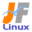Linux Kernel 2.6 Documentation: /usr/src/linux/Documentation/usb/ohci.txt

usb/ohci.txt

ohci \$B%I%i%\$%P\$N@bL@(B [\$B%W%l%\$%s%F%-%9%HHG(B]
• \$B86Cx:n
• \$BK]Lu
• \$B%P!<%8%g%s(B: 2.6.14
• \$BK]LuF|;~(B: 2005/11/12
```

==================================
\$B\$3\$l\$O!"(B
linux-2.6.14/Documentation/usb/ohci.txt \$B\$NOBLu(B
\$B\$G\$9!#(B
\$BK]LuCDBN!'(B JF \$B%W%m%8%'%/%H(B < http://www.linux.or.jp/JF/ >
\$B99?7F|(B \$B!'(B 2005/11/12
\$B86Cx:n\$N?M\$?\$A\$+\$i\$N=uNO\$bo(B OHCI \$B\$r;H\$\$\$^\$9!#(B

Changes since the 2.4 kernel include

2.4 \$B%+!<%M%k\$+\$i\$NJQ99E@\$O!".(B
- \$B99?7!"4JN,2=\$5\$l\$?(B usbcore API \$B\$N%5%]!<%H(B
- \$B%\$%s%?%i%W%HE>Aw\$O!"\$5\$i\$KBg\$-\$/\$G\$-!"BT\$A9TNs\$KF~\$l\$k\$3\$H\$b\$G\$-\$^\$9(B
- \$B\$h\$j>e0L\$N(B "hcd" \$B%U%l!<%`%o!<%/\$r;H\$&\$3\$H\$K\$h\$k!"%3!<%I\$N=L>.(B
- OHCI \$B\$N!"\$\$\$/\$D\$+\$N(B \$BHs(B PCI \$B(B

The "ohci-hcd" driver handles all USB 1.1 transfer types.  Transfers of all
types can be queued.  That was also true in "usb-ohci", except for interrupt
transfers.  Previously, using periods of one frame would risk data loss due
to overhead in IRQ processing.  When interrupt transfers are queued, those
risks can be minimized by making sure the hardware always has transfers to
work on while the OS is getting around to the relevant IRQ processing.

"ohci-hcd" \$B%I%i%\$%P\$O!"\$9\$Y\$F\$NAw\$r07\$\$\$^\$9!#\$9\$Y\$F\$N(B
\$BAw\$OBT\$A9TNs\$KF~\$l\$k\$3\$H\$,\$G\$-\$^\$9!#(B"usb-ohci" \$B\$b!"%\$%s%?%i%W%HE>Aw\$r\$N\$>\$\$\$F(B
\$BF1MM\$KBT\$A9TNs\$r%5%]!<%H\$7\$^\$9!#0JA0\$O!"\$R\$H\$D\$N%U%l!<%`\$GJ#?t\$N<~4|\$r;H\$&(B
\$B\$N\$O!"(BIRQ \$B=hM}Cf\$N%*!<%P!<%X%C%I\$K\$h\$j!"%G!<%?>C<:\$N4m81@-\$,\$"\$j\$^\$7\$?!#(B
\$BJ#?t\$N%\$%s%?%i%W%HE>Aw\$,BT\$A9TNs\$KF~\$l\$i\$l\$?\$H\$-!"(BOS \$B\$,(B IRQ \$B4XO"=hM}\$r\$7\$F\$\$\$k(B
\$B4V\$b!"%O!<%I%&%'%"\$,>o\$KE>Aw=hM}\$r\$9\$k\$N\$,3N.(B
\$B\$K\$G\$-\$^\$9!#(B

- David Brownell
<dbrownell at users dot sourceforge dot net>
```